##### Simultaneous linear equations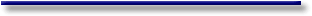# Online Econometrics Textbook - Regression Extensions - Simultaneous linear equations

In simultaneous equation systems there are always feedback structures which make it (almost) impossible to use the easy methods we've described before.

As a matter of fact we have to make a firm distinction between two different kinds of variables: the jointly dependent variables (or endogenous variables), and the predetermined variables (or exogenous variables).

The jointly dependent variables may (but don't have to) be used as dependent and explanatory variables at the same time (in different equations). The predetermined variables however are all of those which are not explicitly explained by other variables in any equation. This means that a "lagged" version of a dependent variable is in fact considered to be exogenous or rather predetermined.

The variance-covariance matrix of the residuals is assumed to be of the form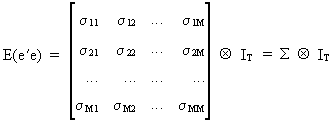(III.III-1)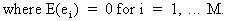The model of the structural equations can be written as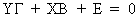(III.III-2)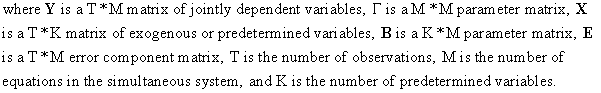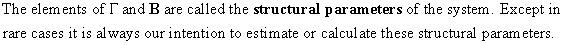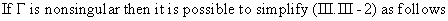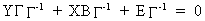(III.III-3)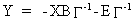(III.III-4)

and thus to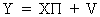(III.III-5)

in order to get the reduced form equations.

Remark that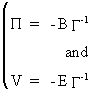(III.III-6)

which are called the reduced form parameters and the reduced form disturbance respectively.

In fact, by transforming a structural form into a reduced form, what one does is to express all jointly dependent variables as a function of all predetermined variables.

The asymptotic assumptions are expressed explicitly as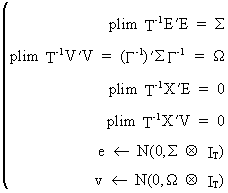(III.III-7)

Since it is our objective to estimate the structural parameters of (III.III-2) we could start naively with OLS estimations to each equation separately (after having normalized the equations)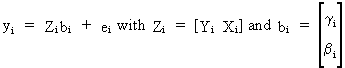(III.III-8)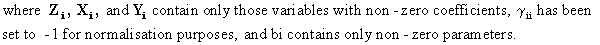Except in rare cases this procedure will not yield unbiased parameter estimations since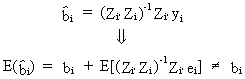(III.III-9)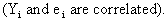Above that, the estimated value of the parameters does not converge in probability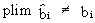(III.III-10)

The results in (III.III-9) and (III.III-10) are known as the least squares bias. It is therefore quite obvious that we have to find other ways of estimating the structural parameters, without bias.

We know that estimating the reduced form with OLS would be at least consistent since the assumptions of (III.III-7) can be used to prove that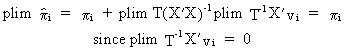(III.III-11)

The OLS estimator will also be unbiased (for estimating the reduced form parameters) if X doesn't contain lagged jointly dependent variables.

Suppose we use (III.III-11) in the context of SUR then we know that this is equivalent to GLS since all the exogenous variables are identical in all equations.

After having used OLS with SUR it is sometimes possible to compute the structural form parameters knowing that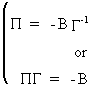(III.III-12)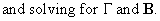This procedure is known as the Indirect Least Squares method (ILS).

When is it possible to find a unique solution with ILS? Answer: only when the equations are exactly identified. If an equation is under identified it is never possible to compute the structural parameters. If however an equation is over identified there are more than one unique solution to (III.III-12), and consequently some special techniques should be used to solve this problem.

Let us have a look at the i-th equation of a structural form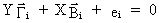(III.III-13)

Since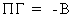(III.III-14)

we know that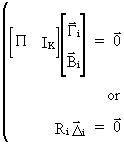(III.III-15)

A necessary and sufficient condition for exact identification is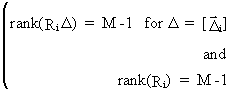(III.III-16)

which is called the rank condition. Here ILS is consistent and efficient.

A condition for over identification is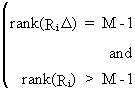(III.III-17)

where ILS is consistent but not efficient.

A condition for under identification is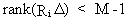(III.III-18)

where estimation with ILS is not possible.

A necessary condition for identification is therefore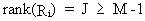(III.III-19)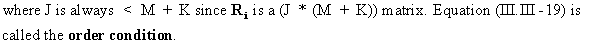Now we consider the problem of estimating the structural parameters of an over identified system by use of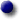the ILS approachthe GLS approach

The ILS approach

A single-equation structural form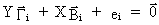(III.III-20)

with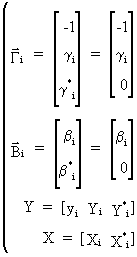(III.III-21)

can be written as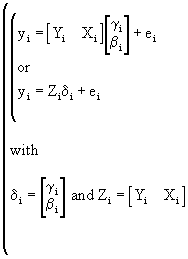(III.III-22)

Since the reduced form parameters may be consistently estimated by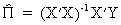(III.III-23)

and since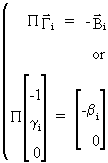(III.III-24)

it follows that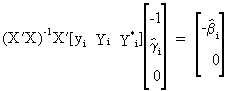(III.III-25)

and by premultiplying by X'X we get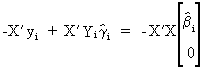(III.III-26)

and thus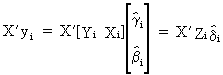(III.III-27)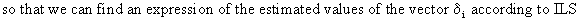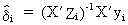(III.III-28)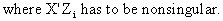The GLS approach

When the model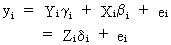(III.III-29)

is premultiplied by X' we obtain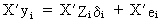(III.III-30)

It can easily be shown, using (III.III-30), that the GLS estimator is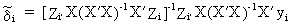(III.III-31)

or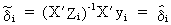(III.III-32)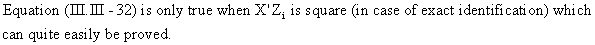To prove consistency, assume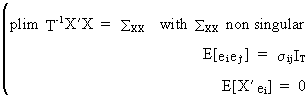(III.III-33)

which can be used to show that(III.III-34)

The consistent Two Stage Least Squares estimator (2SLS) consists of the following two stages

first stage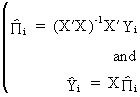(III.III-35)

second stage(III.III-36)

Asymptotically efficient estimators

All the previous estimators are not efficient (in general) since they don't use the information to the full extent. Therefore we describe two Full Information methods: the Tree Stage Least Squares (3SLS), and the Full Information Maximum Likelihood Estimation (FIMLE).

The 3SLS estimator uses the following model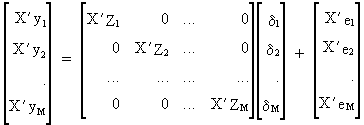(III.III-37)

or simply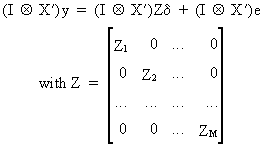(III.III-38)

If X'X/T converges to a nonstochastic limit then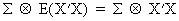(III.III-39)

and accordingly the 3SLS estimator can be deduced as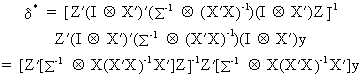(III.III-40)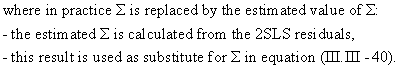If all M equations are identified, the 3SLS estimator is consistent. Above this, the 3SLS method is more efficient than the 2SLS method.

The FIMLE method can be used under the assumption that the distribution of the error terms is known (e.g. normal).

Assume that the errors are jointly normally distributed with a zero mean and covariance matrix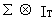(III.III-41)

then the FIMLE method can be applied by means of for instance a numerical iterative algorithm. Some examples of algorithms are: the Grid search method, the Newton-Raphson method, the Gauss-Newton method, the Steepest Descend method, the Marquardt algorithm etc...© 2000-2022 All rights reserved. All Photographs (jpg files) are the property of Corel Corporation, Microsoft and their licensors. We acquired a non-transferable license to use these pictures in this website.
The free use of the scientific content in this website is granted for non commercial use only. In any case, the source (url) should always be clearly displayed. Under no circumstances are you allowed to reproduce, copy or redistribute the design, layout, or any content of this website (for commercial use) including any materials contained herein without the express written permission.

Information provided on this web site is provided "AS IS" without warranty of any kind, either express or implied, including, without limitation, warranties of merchantability, fitness for a particular purpose, and noninfringement. We use reasonable efforts to include accurate and timely information and periodically updates the information without notice. However, we make no warranties or representations as to the accuracy or completeness of such information, and it assumes no liability or responsibility for errors or omissions in the content of this web site. Your use of this web site is AT YOUR OWN RISK. Under no circumstances and under no legal theory shall we be liable to you or any other person for any direct, indirect, special, incidental, exemplary, or consequential damages arising from your access to, or use of, this web site.

Contributions and Scientific Research: Prof. Dr. E. Borghers, Prof. Dr. P. Wessa
Please, cite this website when used in publications: Xycoon (or Authors), Statistics - Econometrics - Forecasting (Title), Office for Research Development and Education (Publisher), http://www.xycoon.com/ (URL), (access or printout date).